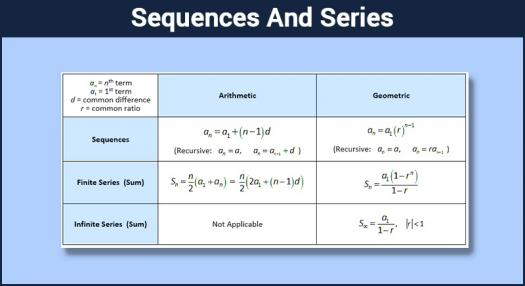# Sequences & Series Assessment Test

10 Questions | Total Attempts: 137SettingsSequence is an order of numbers such that the difference of any two successive members is a constant, while series is the sum of the various numbers, or elements of a sequence.

Related Topics
• 1.
The first term of a G.P is twice its common ratio. Find the sum of the first two terms of the progression if its sum to infinity is...
• A.

8/5

• B.

8/3

• C.

72/25

• D.

56/9

• 2.
The third term of an A.P is 4x-2y and the 9th term is 10x-8y. Find the common difference.
• A.

19x-17y

• B.

x-y

• C.

8x-4y

• D.

2x

• 3.
Evaluate (-1/2 - 1/4 + 1/8 - 1/16 +... ) -1.
• A.

2/3

• B.

0

• C.

-2/3

• D.

-1

• 4.
The sixth term of an arithmetical progression is half of its twelfth term. The first term is equal to...
• A.

The common difference

• B.

Half of the common difference

• C.

Zero

• D.

Double of the common difference

• 5.
If the nth term of an A.P is five times the 5th term, find the relationship between a and d.
• A.

A+2d=0

• B.

2a+d=0

• C.

3a+5d=0

• D.

A+3d=0

• 6.
If the harmonic mean and geometric mean of two numbers a and b are 4 and 3 2 respectively then the interval [a, b] = ___.
• A.

[3, 6]

• B.

[2, 7]

• C.

[4, 5]

• D.

[1, 8]

• 7.
If for the triangle whose perimeter is 37 cm and length of sides are in G.P. also the length of the smallest side is 9 cm, then length of remaining two sides are ___ and __.
• A.

12; 16

• B.

14; 14

• C.

10; 18

• D.

15; 13

• 8.
If 2, b, c, 23 are in a GP, then (b - c)2 + (c - 2)2 + (23 - b)2 = ____.
• A.

625

• B.

525

• C.

441

• D.

428

• 9.
The coefficient of x8 in the product (x+1) (x+2) (x+3) ... (x+10) is...
• A.

1024

• B.

1300

• C.

1320

• D.

1360

• 10.
The series 1. 1! + 2. 2! + 3. 3! + ... + n. n! = ?
• A.

(n + 1)! -n

• B.

(n + 1)! -1

• C.

N! -1 + n

• D.

N! +1 -n